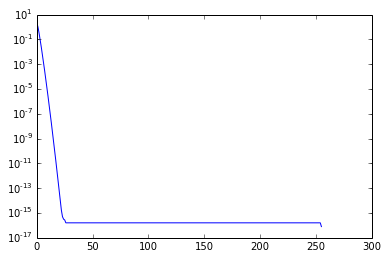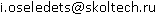## Cross approximation: intro

26 февраля 2014

### Skeleton decomposition

Cross approximation and skeleton decomposition are one of the basic concepts in our research. It is all about low-rank matrices which appear in many different applications: integral equations, tensor approximations and many others. Cross approximation is simple and elegant, but it is not widely known as it should be. Suppose that an $$n \times m$$ matrix $$A$$ has rank $$r$$. Then it can be exactly recovered from $$r$$ columns and $$r$$ rows as

$$A = C \widehat{A}^{-1} R,$$

where $$C$$ are $$r$$ columns of $$A$$, $$R$$ are $$r$$ rows of $$A$$ and $$\widehat{A}$$ is a submatrix on their intersection. That means, that only $$(n + m) r$$ elements are required. What about the stability of this decomposition? Suppose $$A$$ can be approximated with low rank only approximately:

$$A = A_r + E,$$

with $$\Vert E \Vert = \varepsilon$$. How to select an good submatrix. A good guide is the maximum volume : among all $$r \times r$$ submatrices select the one that has largest volume, i.e. the maximum absolute value of the determinant. Then, the corresponding approximation

$$\Vert A - A_{maxvol} \Vert_C \leq (r + 1) \sigma_{r + 1},$$

where $$\sigma_{r + 1}$$ is the $$(r + 1)$$-th singular value of $$A$$ and the error is measured in the Chebyshev norm (maximum absolute value).

### Cross approximation

A good question is how to compute (quasi)-optimal submatrices. One the possibility is the cross approximation algorithm, which is equivalent to the Gaussian elimination. The steps of the algorithm are simple:

1. Find some pivot $$(i', j')$$
2. Subtract a rank-$$1$$ cross from the matrix:
$$A_{ij} := A_{ij} - \frac{A_{i j'}A_{i' j}}{A_{i' j'}}.$$
3. Cycle until the norm of $$A$$ is small enough.

Different pivoting strategies are possible. The full pivoting assumes that $$(i', j')$$ maximizes the absolute value of the residue. This involves $$\mathcal{O}(nm)$$ complexity and is unacceptable. Partial pivoting can be different: from the simplest row pivoting scheme to rook schemes and random sampling.

### Maximum-volume

Maximum-volume principle is the cornerstone of the low-rank approximation algorithms. Although it is often claimed that maximum-volume submatrix is hard to compute, there are very efficient algorithms for doing that. Maybe the most promiment one is the algorithm that computes a maximum-volume submatrix in a tall $$n \times r$$ matrix. It is an iterative algorithm: it starts from a non-singular $$r \times r$$ submatrix and then substitutes one row at a step (greedy approach). For this problem, the convergence is very fast. There are few tricks to make it really efficient. The efficient implementations of the maxvol algorithm are available both in the MATLAB and Python version of the TT-Toolbox.

In :
import numpy as np
import tt
from tt.maxvol import maxvol
n = 256
a = np.zeros((n, n))
""" Hilbert matrix --- classic low-rank example """
for i in xrange(n):
for j in xrange(n):
a[i, j] = 1.0 / (i + j + 1)
u, s, v = np.linalg.svd(a)
semilogy(s)

Out:
[<matplotlib.lines.Line2D at 0x10cc69b50>]Computing the maxvol-skeleton approximation

In :
for r in [1, 5, 10, 15]:
u1 = u[:, :r]
v1 = v[:r, :]
v1 = v1.T
s1 = s[:r]
er_svd = np.linalg.norm(a - np.dot(u1, np.dot(np.diag(s1), v1.T)))
""" Computing maxvol indices """
indu = sort(maxvol(u1))
indv = sort(maxvol(v1))
C = a[:, indv]
C = np.linalg.solve(C[indu, :].T, C.T).T # Computing CC^{-1}
R = a[indu, :]

er_skel = np.linalg.norm(a - np.dot(C, R))

print 'SVD er:', er_svd, 'Skeleton er:', er_skel

SVD er: 1.05910918542 Skeleton er: 1.62572489102
SVD er: 0.00594784107656 Skeleton er: 0.0181112433106
SVD er: 2.76537410866e-06 Skeleton er: 5.17080332494e-06
SVD er: 5.96043397807e-10 Skeleton er: 1.55873142365e-09


News

 01/12/2015 New paper in SIMAX 05/11/2015 New paper in J. Comp. Phys 04/11/2015 New paper in J. Chem. Phys 07/09/2015 Anton Sukhinov leaves the group 29/08/2015 MMMA-2015

## Contact

We are located at the 2-nd floor of the new "Technopark-3” building in Skolkovo (few kilometers outside Moscow Ring Road). The building is accessible from Skolkovo Road (Сколковское шоссе) and Minskoe Highway (Минское шоссе).

email: